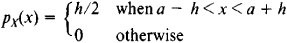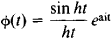# Uniform Distribution

(redirected from Uniform random variable)

## uniform distribution

[′yü·nə‚fȯrm ‚di·strə′byü·shən]
(statistics)
The distribution of a random variable in which each value has the same probability of occurrence. Also known as rectangular distribution.
McGraw-Hill Dictionary of Scientific & Technical Terms, 6E, Copyright © 2003 by The McGraw-Hill Companies, Inc.
The following article is from The Great Soviet Encyclopedia (1979). It might be outdated or ideologically biased.

## Uniform Distribution

a special type of probability distribution of a random variable X that takes on values in the interval (a - h, a + h). A uniform distribution is characterized by the probability density functionThe mathematical expectation is EX = a, the variance is D X= h2/3, and the characteristic function isBy means of a linear transformation the interval (a - h, a + h) can be made to correspond to any given interval. Thus, the variable Y = (X - a + h)/2h is uniformly distributed over the interval (0, 1). Suppose the variables Y1, Y2,.…, Yn are uniformly distributed over the interval (0, 1). When their sum is normalized by the mathematical expectation n/2 and the variance n/12, the distribution law of the normalized sum rapidly approaches a normal distribution as n increases. In fact, the approximation is often sufficient for practical applications even when n = 3.

References in periodicals archive ?
Let U [member of] U(0,1) be a Standard Uniform random variable.
Equal distribution function of the uniform random variable U:
To this end, suppose that [D.sub.m] is a discrete uniform random variable on the first m positive integers, so that its mass function is given by P([D.sub.m] = d) = 1/m for d = 1,2, ..., m, and P([D.sub.m] = d) = 0 otherwise.
Use can then be made of the continuous uniform random variable V with probability density function given by
* Let [mu] be a distribution on R with cumulative distribution function F .If U is uniform on [0,1] then the law of [F.sup.-1](U) is where [F.sup.-1](u) = inf {x | F(x) [greater than or equal to] u} is the right continuous inverse of F; hence to simulate any distribution a uniform random variable on [0,1] is sufficient.
Let U [epsilon] U(0,1) be a Standard Uniform random variable.
This example deals with 48 uncertain parameters, which are considered as uniform random variables having an uncertainty of 20% around their nominal values given in Fig.
The distribution of the sum of uniform random variables that may have differing domains is found in [18-21].
uniform random variables on [0,1], we conjectured that the number of proper hyperedges in an optimal solution is expected to be n for the hypergraph ([G.sub.2,2n], and showed computational results supporting this conjecture.
In order to establish an approach for evaluating the system reliability of such systems, we assume the loss factors are continuous uniform random variables and generate N uniformly distributed pseudo-random numbers in the interval [0, 1].
Hence N = (1.039921 + 1.006469)/2 = 1.023195 is the average consumption of uniform random variables in Algorithm NA.
The size of an object is therefore a product of independent uniform random variables times X.

Site: Follow: Share:
Open / Close